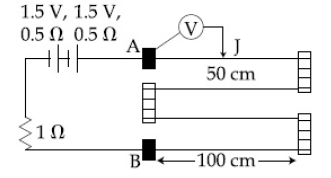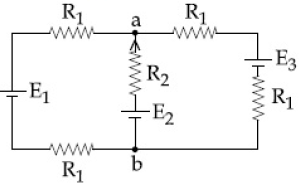# Q&A - Ask Doubts and Get Answers

Sort by :
Clear All
Q

In a conductor , if the number of conduction electron per unit volume is   and mean free time is  ( femto second ) , it's approximate resistivity is :

• Option 1)

• Option 2)

• Option 3)

• Option 4)

Resistivity - - wherein It is independent of shape and size of the body i.e.  and      we know that , Option 1) Option 2) Option 3) Option 4)

Space between two concentric conducting spheres of radii a and  b (b>a) is filled with a medium of resistivity . The resistance between the two spheres will be :

• Option 1)

• Option 2)

• Option 3)

• Option 4)

Take a shell at distnce r from centre and find out its Resistance .   Option 1) Option 2) Option 3) Option 4)

In an experiment , the resistance of a material is plotted as a function of temperature (in some range).

As shown in the figure, it is a staright line.One may conclude that:

• Option 1)

• Option 2)

• Option 3)

• Option 4)

Dependence of Resistance - i) Length of conductor -    ii) Area of cross-section  -   iii) Temperature (for conductor) -     Let   y = mx + c Or where  is constant  So, correct option is (2) which is    Option 1) Option 2) Option 3) Option 4)

Which of the following combinations has the dimension of electrical resistance (  is the permittivity of vacuum and  is the permeability of vacuum) ?

• Option 1)

• Option 2)

• Option 3)

• Option 4)

Unit and Dimension - Ohm.mt - S.I Unit   Dimensions - wherein specific resistance     Dimension of  Dimension of  Check option (1)  Option 1) Option 2) Option 3) Option 4)

The resistive network shown below is connected to a D.C. source of 16 V. The power consumed by the network is 4 Watt. The value of R is :• Option 1)

• Option 2)

• Option 3)

• Option 4)

Heat developed in a resistor - When a steady current flows through a resistance R for time t , the loss in electric potential energy appears as increased thermal energy(Heat H) of resistor and  The power devoleped =       (from Ohm's law) - wherein Unit of heat is joule (J) Unit of power is watt (W)     Option 1) Option 2) Option 3) Option 4)

A galvanometer of resistance  has 50 divisions on its scale and has sensitivity of  division. It is to be converted to a voltmeter with three ranges, of  and . The appropriate circuit to do so is :

• Option 1)• Option 2)• Option 3)• Option 4)Conversion of galvanometer into voltameter - Connected a large Resistance   in series - wherein     option (2) can not ever be appropriate circuit because we can not measure high voltage i.e 20V before smaller voltage i.e 2V, because if the higher voltage is at the end then the resistance is large and hence more range. Now checking option (1), (2), & (3) option (1) is...

A current of 5 A  passes through a copper conductor ( Resistivity = )

of radius of cross-section 5mm. Find the mobility of the charges if their drift velocity is .

• Option 1)

• Option 2)

• Option 3)

• Option 4)

Mobility - - wherein  Mobility  Drift velocity     Option 1) Option 2) Option 3) Option 4)

In the given meter bridge experiment,the circuit diagram

and the corresponding observation table are shown in fig.Which of the reading is inconsistent?

• Option 1)

3

• Option 2)

2

• Option 3)

4

• Option 4)

1

Meter bridge - It works on the principle of wheat stone bridge -     Meter bridge - - wherein    For meter bridge balanced condition =>  for (1)  for (2)  for (3)  for (4)  From this , we can conclude that observation (4) is inconsistent. Option 1) 3 Option 2) 2 Option 3) 4 Option 4) 1

In the given circuit , an ideal voltmeter connected across the

resistance reads 2V. The internal resistance r , of each cell is :• Option 1)• Option 2)• Option 3)• Option 4)In series Grouping - - wherein  Equivalent Resistance     In parallel Grouping - -     So, V = 6 x i = 2        => =>  Option 1) Option 2) Option 3) Option 4)

A moving coil galvanometer allows a full  scale current of  A. A series resistance  of 200 is required to convert the above galvanometer into an voltmeter of range0-5 V . Therefore the value of shunt resistance required to convert the above galvanometer into an ammeter of range0-10 mA is :

• Option 1)

• Option 2)

• Option 3)

• Option 4)

Conversion of galvanometer into voltameter - Connected a large Resistance   in series - wherein     (R+G) I = V =>   G=300 Option 1) Option 2) Option 3) Option 4)

A moving coil galvanometer, having a resistance G, produces full-scale deflection when a current  flows through it. This galvanometer can be converted into (i) an ammeter of range 0 to  by connecting a shunt resistance  to it and (ii) into a voltmeter of range 0 to  by connecting a series resistance  to it. Then,

• Option 1)

and

• Option 2)

and

• Option 3)

and

• Option 4)

Conversion of galvanometer into voltameter - Connected a large Resistance   in series - wherein                                                                              Option 1)  and          Option 2)  and  Option 3)  and  Option 4)

One kg of water, at , is heated in an electric kettle whose heating element has a mean (temperature averaged) resistance of . The rms voltage in the mains is 200 V. Ignoring heat loss from the kettle, time taken for water to evaporate fully, is close to :

[ Specific heat of water =, Latent heat of water = 2260 kJ/kg]

• Option 1)

16 minutes

• Option 2)

22 minutes

• Option 3)

3 minutes

• Option 4)

10 minutes

Heat developed in a resistor - When a steady current flows through a resistance R for time t , the loss in electric potential energy appears as increased thermal energy(Heat H) of resistor and  The power devoleped =       (from Ohm's law) - wherein Unit of heat is joule (J) Unit of power is watt (W)       Option 1) 16 minutes  Option 2) 22 minutes  Option 3) 3 minutes  Option 4) 10 minutes

In the figure shown, what is the current (in ampere) drawn from the battery? you are given:• Option 1)

13/24

• Option 2)

7/18

• Option 3)

9/32

• Option 4)

20/3

Option 1)13/24Option 2)7/18Option 3)9/32Option 4)20/3

A cell of internal resistance r drives current through an external resistance R. The power delivered by the cell to the external resistance will be maximum when:

• Option 1)

R=0.001 r

• Option 2)

R=1000 r

• Option 3)

R=2r

• Option 4)

R=r

P across  For maximum=  R=r   Option 1) R=0.001 r Option 2) R=1000 r Option 3) R=2r Option 4) R=r

In the circuit shown, a four-wire potentiometer is made of a 400 cm long wire, which extends between A and B. The resistance per unit length of the potentiometer wire is . if an ideal voltmeter is connected as shown with jockey J at 50 cm from end A, the expected reading of the voltlmeter will be:• Option 1)

0.50 V

• Option 2)

0.75 V

• Option 3)

0.25 V

• Option 4)

0.20 V

Option 1) 0.50 V Option 2) 0.75 V Option 3) 0.25 V Option 4) 0.20 V

The resistance of a galvanometer is  and the maximum current which can be passed through it is   . What resistance must be connected to it in order to convert it into an ammeter of range  ?

• Option 1)

• Option 2)

• Option 3)

• Option 4)

For converting galvanometer into ammeter we must add a short resistance  Given, Option 1) Option 2) Option 3) Option 4)

A metal wire of resistance   is elongated to make a uniform wire of double its previous length . This new wire is now bent and the ends joined to make a circle . If two points on this circle make an angle at the centre , the equivalent resistance between these two points will be :

• Option 1)

• Option 2)

• Option 3)

• Option 4)

After elongation Vol of wire initial = vol of wire  final Option 1) Option 2)   Option 3) Option 4)

A moving coil galvanometer has resistance  and it indicates full deflaction at  current. A voltmeter is made using this galvanometer and a  resistance. The maximum voltage , that can be measured using this voltmeter,will be close to :

• Option 1)

• Option 2)

• Option 3)

• Option 4)

Given                   Series resistance                                Option 1)Option 2)Option 3)Option 4)

A wire of resistance  is bent to form a square  as shown in the figure. The effective resistance between  and  is :(  is mid-point of arm CD)• Option 1)

• Option 2)

• Option 3)

• Option 4)

distribution of Resistance (R) in square                                    Option 1) Option 2)   Option 3) Option 4)

For the circuit shown, with and the potential difference between the points 'a' and 'b' is approximately(in V) :• Option 1)

• Option 2)

• Option 3)

• Option 4)

For parallal combination of cells Option 1)Option 2)Option 3)  Option 4)
Exams
Articles
Questions